﻿ Graphs of Sine and Cosine

# GRAPHS OF SINE AND COSINE

• PRACTICE (online exercises and printable worksheets)

The graphs of the sine and cosine functions are shown below.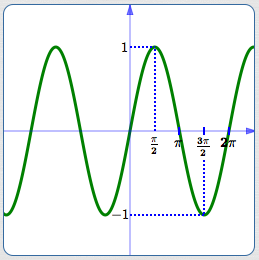graph of $y = \sin x$graph of $y = \cos x$

## Where do these graphs come from?

The sine function gives the $y$-values of points on the unit circle.
The cosine function gives the $x$-values of points on the unit circle.

Since the unit circle has radius $\,1\,$, all its points have coordinates between $\,-1\,$ and $\,1\,$.
That's why both graphs (sine and cosine) are trapped between $\,y = -1\,$ and $\,y = 1\,$.

 Here's a way you can visualize the graph of the sine function: Put your finger at the point $\,(1,0)\,$ on the unit circle. Twirl it around the circle counter-clockwise (start UP).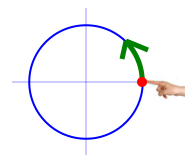### The sine function tracks your finger's up/down motions: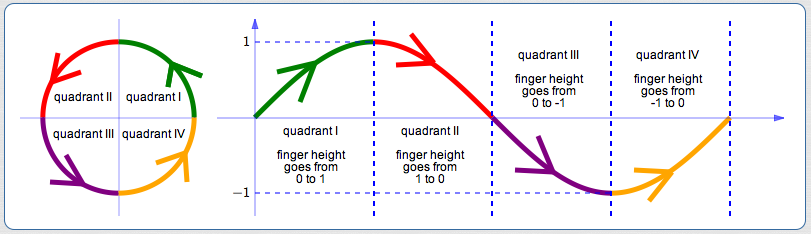### A higher level of understanding for the sine function:

In Radian Measure, we ‘wrap’ the real number line around the unit circle.
In this way, every real number is associated with a point (called the terminal point) and a corresponding angle on the unit circle.
The real number is then the radian measure of this angle!

 Wrap the real number linearound the unit circle!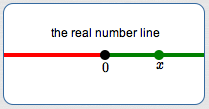Thus, every real number $\,x\,$ ...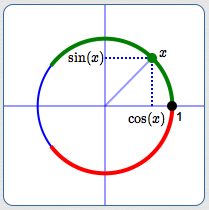... is associated with a point on the unit circle ...... and a corresponding angle.

In The Unit Circle Approach to Trigonometry, we saw that $\,\sin(x)\,$ is the $y$-value of the terminal point:
 As $\,x\,$ goes from $\,0\,$ to $\,\frac{\pi}{2}\,$, $\,\sin x\,$ goes from $\,0\,$ to $\,1\,$. (Remember: $\,\frac{\pi}{2}\,$ radians is $\,90^\circ\,$)As $\,x\,$ goes from $\,\frac{\pi}{2}\,$ to $\,\pi\,$, $\,\sin x\,$ goes from $\,1\,$ back to $\,0\,$. (Remember: $\,\pi\,$ radians is $\,180^\circ\,$)### The cosine function:

The entire discussion can be repeated to understand the cosine—except that it gives the $x$-values of the points, not the $y$-values!
Here's how one of the graphics above would be adjusted to focus attention on the $x$-value:## Important Characteristics of the Graphs

Properties of both sine and cosine:

• domain is $\Bbb R$
(Recall that $\,\Bbb R\,$ represents the set of real numbers.)
• range is the interval $[-1,1]$
• the period is $\,2\pi\,$
 sine has period $\,2\pi\,$: $\sin(x+2\pi) = \sin x\,$ for all real numbers $\,x\,$ More generally: $\sin(x+2\pi k) = \sin x\,$   for all integers $\,k\,$ and all real numbers $\,x\,$ cosine has period $\,2\pi\,$: $\cos(x+2\pi) = \cos x\,$ for all real numbers $\,x\,$ More generally: $\cos(x+2\pi k) = \cos x\,$   for all integers $\,k\,$ and all real numbers $\,x\,$

## Two Trigonometric Identities

Take the sine curve and shift it $\,\frac{\pi}{2}\,$ units to the left—it turns into the cosine curve: $$\sin(x + \frac{\pi}{2}) = \cos x$$ Take the cosine curve and shift it $\,\frac{\pi}{2}\,$ units to the right—it turns into the sine curve: $$\cos(x - \frac{\pi}{2}) = \sin x$$Thus, we have two new trigonometric identities!
For all real numbers $\,x\,$:

• $\displaystyle\sin(x + \frac{\pi}{2}) = \cos x$
• $\displaystyle\cos(x - \frac{\pi}{2}) = \sin x$

Master the ideas from this section
like $\,y=a \sin k(x±b)\,$ and $\,y = a \cos(kx±B)$